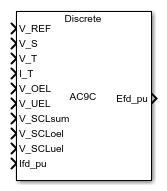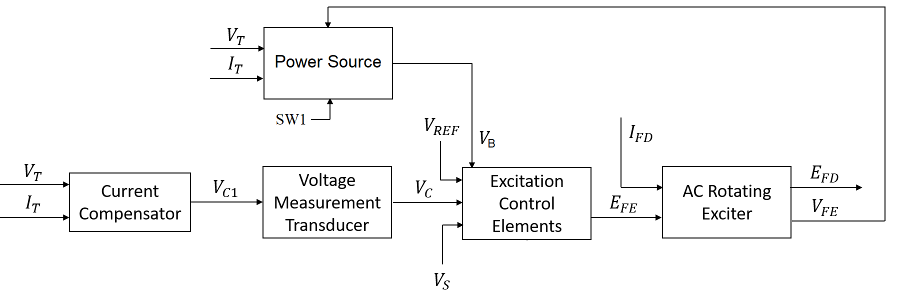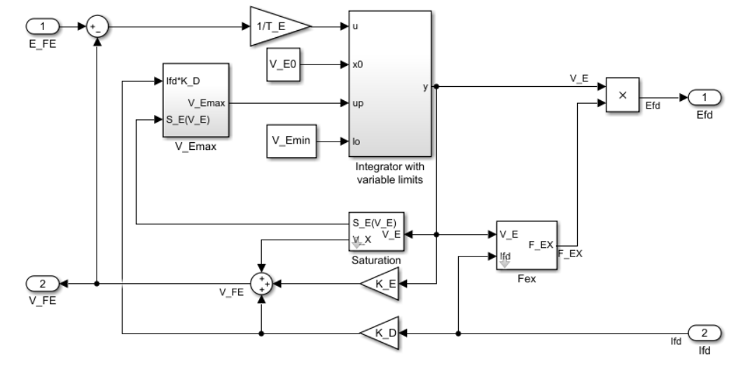# SM AC9C

Discrete-time or continuous-time synchronous machine AC9C excitation system including an automatic voltage regulator and an exciter

Since R2023a

•Libraries:
Simscape / Electrical / Control / SM Control

## Description

The SM AC9C block implements a synchronous machine type AC9C excitation system model in conformance with Std IEEE 421.5-2016 .

Use this block to model the control and regulation of the field voltage of a synchronous machine that operates as a generator using an AC rotating exciter.

Switch between continuous and discrete implementations of the block by using the Sample time (-1 for inherited) parameter. To configure the integrator for continuous time, set the Sample time (-1 for inherited) parameter to `0`. To configure the integrator for discrete time, set the Sample time (-1 for inherited) parameter to a positive scalar. To inherit the sample time from an upstream block, set the Sample time (-1 for inherited) parameter to `-1`.

The SM AC9C block comprises five major components:

• The Current Compensator component modifies the measured terminal voltage as a function of the terminal current.

• The Voltage Measurement Transducer component simulates the dynamics of a terminal voltage transducer using a low-pass filter.

• The Excitation Control Elements component compares the voltage transducer output with a terminal voltage reference to produce a voltage error value. The component then passes this value through a voltage regulator to produce the exciter field voltage.

• The AC Rotating Exciter component models the AC rotating exciter, which produces a field voltage that is applied to the controlled synchronous machine. The block also feeds the exciter field current (VFE) back to the excitation system.

• The Power Source component models the dependency of the power source for the controlled rectifier from the terminal voltage.

This diagram shows the structure of the AC9C excitation system model:In the diagram:

• VT and IT are the measured terminal voltage and current of the synchronous machine, respectively.

• VC1 is the current-compensated terminal voltage.

• VC is the filtered, current-compensated terminal voltage.

• VREF is the reference terminal voltage.

• VS is the power system stabilizer voltage.

• SW1 is the power source switch that you specify for the controlled rectifier.

• VB is the exciter field voltage.

• EFE and VFE are the exciter field voltage and current, respectively.

• EFD and IFD are the field voltage and current, respectively.

### Current Compensator and Voltage Measurement Transducer

The block models the current compensator by using this equation:

`${V}_{C1}={V}_{T}+{I}_{T}\sqrt{{R}_{C}^{2}+{X}_{C}^{2}},$`

where:

• RC is the load compensation resistance.

• XC is the load compensation reactance.

The block implements the voltage measurement transducer as a Low-Pass Filter block with the time constant TR. Refer to the documentation for the Low-Pass Filter block for information about the exact discrete and continuous implementations.

### Excitation Control Elements

This diagram shows the structure of the excitation control elements:In the diagram:

• The Summation Point Logic subsystem models the summation point input location for the overexcitation limiter (OEL), underexcitation limiter (UEL), and stator current limiter (SCL). For more information about using limiters with this block, see Field Current Limiters.

• The PID subsystem models a PID controller that functions as a control structure for the automatic voltage regulator. The minimum and maximum anti windup saturation limits for the block are VPIDmin and VPIDmax, respectively.

• The Take-over Logic subsystem models the take-over point input location for the OEL, UEL, SCL and PSS voltages. For more information about using limiters with this block, see Field Current Limiters.

• The PI_R subsystem models a PI controller that functions as a control structure for the field current regulator. The minimum and maximum anti windup saturation limits for the block are VAmin and VAmax, respectively.

• The top Low-Pass Filter block models the major dynamics of the controlled rectified bridge. KA is the controlled rectifier bridge equivalent gain and TA is the major time constant of the controlled rectifier bridge. The minimum and maximum anti windup saturation limits for the block are VRmin and VRmax, respectively.

• The bottom Low-Pass Filter block models the rate feedback path for the stabilization of the excitation system. KF and TF are the gain and time constants of this system, respectively. See the documentation for the Low-Pass Filter block for information about the discrete and continuous implementations.

• The Power state logic subsystem supports the selection of the power stage type, which can be a thyristor or a chopper converter. If you set the Power stage type selector, S_CT parameter to `Thyristor bridge`, it represents a thyristor converter. If you set the Power stage type selector, S_CT parameter to ```Chopper converter```, it represents a chopper converter. The subsystem sums the voltage regulator command signal VR to the exciter field voltage VB. For more information about the logical switch for the power source of the controlled rectifier and about the power state logic subsystem, see Power Source and Power State Logic.

### Field Current Limiters

You can use different types of field current limiter to modify the output of the voltage regulator under unsafe operating conditions:

• Use an overexcitation limiter to prevent overheating of the field winding due to excessive field current demand.

• Use an underexcitation limiter to boost field excitation when it is too low, which risks desynchronization.

• Use a stator current limiter to prevent overheating of the stator windings due to excessive current.

Attach the output of any of these limiters at one of these points:

• Summation point — Use the limiter as part of the automatic voltage regulator (AVR) feedback loop.

• Take-over point — Override the usual behavior of the AVR.

If you are using the stator current limiter at the summation point, use the input VSCLsum. If you are using the stator current limiter at the take-over point, use the overexcitation input VOELscl, and the underexcitation input VUELscl.

### AC Rotating Exciter

This diagram shows the structure of the AC rotating exciter:In the diagram:

• The exciter field current VFE is the sum of three signals:

• The nonlinear function Vx models the saturation of the exciter output voltage.

• The proportional term KE models the linear relationship between the exciter output voltage and the exciter field current.

• The subsystem models the demagnetizing effect of the load current on the exciter output voltage using the demagnetization constant KD in the feedback loop.

• The Integrator with variable limits subsystem integrates the difference between EFE and VFE to generate the exciter alternator output voltage VE. TE is the time constant for this process.

• The nonlinear function FEX models the exciter output voltage drop from the rectifier regulation. This function depends on the constant KC, which itself is a function of commutating reactance.

• The parameters VEmin and VFEmax model the lower and upper limits of the rotating exciter.

### Power Source and Power State Logic

You can use different power source representations for the controlled rectifier by setting the Power source selector SW1 parameter value. To derive the power source for the controlled rectifier from the terminal voltage, set the Power source selector SW1 parameter to ```Position A: power source derived from terminal voltage```. To specify that the power source is independent of the terminal voltage, set the Power source selector SW1 parameter to ```Position B: power source independent from the terminal conditions```.The Power state logic subsystem supports the selection of the power stage type, which can be a thyristor or a chopper converter. If you set the Power stage type selector, S_CT parameter to ```Thyristor bridge```, the Power state logic subsystem represents a thyristor converter. If you set the Power stage type selector, S_CT parameter to `Chopper converter`, the Power state logic subsystem represents a chopper converter. The value of the voltage regulator command signal VR depends on the Voltage limit, V_lim1 (pu) and Voltage limit, V_lim2 (pu) parameters and on the VCT, VFW, and VAVR signals according to this logic:

```if S_CT ~= 0 % Thyristor bridge V_R = V_CT else % Chopper converter if V_AVR > V_lim1 V_R = C_T else if V_AVR > V_lim2 V_R = 0 else V_R = -V_FW end end end```## Ports

### Input

expand all

Voltage regulator reference set point, in per-unit representation, specified as a scalar.

Data Types: `single` | `double`

Input from the power system stabilizer, in per-unit representation, specified as a scalar.

Data Types: `single` | `double`

Terminal voltage magnitude, in per-unit representation, specified as a scalar.

Data Types: `single` | `double`

Terminal current magnitude, in per-unit representation, specified as a scalar.

Data Types: `single` | `double`

Input from the overexcitation limiter, in per-unit representation, specified as a scalar.

#### Dependencies

• To ignore the input from the overexcitation limiter, set Alternate OEL input locations (V_OEL) to `Unused`.

• To use the input from the overexcitation limiter at the summation point, set Alternate OEL input locations (V_OEL) to `Summation point`.

• To use the input from the overexcitation limiter at the take-over point, set Alternate OEL input locations (V_OEL) to `Take-over`.

Data Types: `single` | `double`

Input from the underexcitation limiter, in per-unit representation, specified as a scalar.

#### Dependencies

• To ignore the input from the underexcitation limiter, set Alternate UEL input locations (V_UEL) to `Unused`.

• To use the input from the underexcitation limiter at the summation point, set Alternate UEL input locations (V_UEL) to `Summation point`.

• To use the input from the underexcitation limiter at the take-over point, set Alternate UEL input locations (V_UEL) to `Take-over`.

Data Types: `single` | `double`

Input from the stator current limiter when using the summation point, in per-unit representation, specified as a scalar.

#### Dependencies

• To ignore the input from the stator current limiter, set Alternate SCL input locations (V_SCL) to `Unused`.

• To use the input from the stator current limiter at the summation point, set Alternate SCL input locations (V_SCL) to `Summation point`.

Data Types: `single` | `double`

Input from the stator current limiter to prevent field overexcitation when using the take-over point, in per-unit representation, specified as a scalar.

#### Dependencies

• To ignore the input from the stator current limiter, set Alternate SCL input locations (V_SCL) to `Unused`.

• To use the input from the stator current limiter at the take-over point, set Alternate SCL input locations (V_SCL) to `Take-over`.

Data Types: `single` | `double`

Input from the stator current limiter to prevent field underexcitation when using the take-over point, in per-unit representation, specified as a scalar.

#### Dependencies

• To ignore the input from the stator current limiter, set Alternate SCL input locations (V_SCL) to `Unused`.

• To use the input from the stator current limiter at the take-over point, set Alternate SCL input locations (V_SCL) to `Take-over`.

Data Types: `single` | `double`

Measured per-unit field current of the synchronous machine, specified as a scalar.

Data Types: `single` | `double`

### Output

expand all

Per-unit field voltage to apply to the field circuit of the synchronous machine, returned as a scalar.

Data Types: `single` | `double`

## Parameters

expand all

### General

Initial per-unit voltage to apply to the field circuit of the synchronous machine.

Initial per-unit voltage to apply to the terminal.

#### Dependencies

To enable this parameter, in the Exciter section, set Power source selector SW1 to ```Position A: power source derived from terminal voltage```.

Initial per-unit current to apply to the terminal.

Time between consecutive block executions. During execution, the block produces outputs and, if appropriate, updates its internal state. For more information, see What Is Sample Time? and Specify Sample Time.

For inherited discrete-time operation, set this parameter to `-1`. For discrete-time operation, set this parameter to a positive integer. For continuous-time operation, set this parameter to `0`.

If this block is in a masked subsystem or a variant subsystem that supports switching between continuous operation and discrete operation, promote this parameter to ensure correct switching between the continuous and discrete implementations of the block. For more information, see Promote Block Parameters on a Mask.

### Pre-Control

Resistance used in the current compensation system. Set this parameter and Reactance component of load compensation, X_C (pu) to `0` to disable current compensation.

Reactance used in the current compensation system. Set this parameter and Resistive component of load compensation, R_C (pu) to `0` to disable current compensation.

Equivalent time constant for the voltage transducer filtering.

### Control

Per-unit proportional gain of the voltage regulator.

Per-unit integral gain of the voltage regulator.

Derivative gain of the voltage regulator.

Equivalent lag time constant for the derivative channel of the PID controller.

Maximum admissible per-unit output of the PID regulator.

Minimum admissible per-unit output of the PID regulator.

Per-unit proportional gain of the field current regulator.

Per-unit integral gain of the field current regulator.

Maximum per-unit current regulator output.

Minimum per-unit current regulator output.

Gain of the controlled rectifier bridge.

Time constant of the controlled rectifier bridge.

Maximum per-unit output of the rectifier bridge.

Minimum per-unit output of the rectifier bridge.

Per-unit field current feedback gain of the exciter.

Feedback time constant for the stabilization of the excitation system.

Per-unit free wheel feedback gain of the exciter.

Maximum per-unit free wheel feedback.

Minimum per-unit free wheel feedback.

Option to select the power stage type. If you set the Power stage type selector, S_CT parameter to `Thyristor bridge`, the power stage represents a thyristor converter. If you set the Power stage type selector, S_CT parameter to ```Chopper converter```, the power stage represents a chopper converter.

First automatic voltage regulator limit for the calculation of the voltage regulator command signal.

Second automatic voltage regulator limit for the calculation of the voltage regulator command signal.

Location of the overexcitation limiter input, specified as one of these options:

• `Summation point`V_OEL is an input of the Summation Point Logic subsystem.

• `Take-over`V_OEL is an input of the Take-over Logic subsystem.

Location of the underexcitation limiter input, specified as one of these options:

• `Summation point`V_UEL is an input of the Summation Point Logic subsystem.

• `Take-over`V_UEL is an input of the Take-over Logic subsystem.

Location of the stator current limiter input, specified as one of these options:

• `Summation point` — Use the V_SCLsum input port.

• `Take-over` — Use the V_SCLoel and V_SCLuel input ports.

### Exciter

Proportional constant for the exciter field.

Time constant for the exciter field.

Diode bridge loading factor. This value is proportional to the commutating reactance.

Demagnetization factor related to the exciter alternator reactances.

Exciter output voltage for the first saturation factor.

Saturation factor for the first exciter.

Exciter output voltage for the second saturation factor.

Saturation factor for the second exciter.

Maximum per-unit field current limit reference.

Minimum per-unit exciter voltage output.

Per-unit potential circuit gain coefficient.

Potential circuit phase angle, in degrees.

#### Dependencies

To enable this parameter, set Power source selector SW1 to ```Position A: power source derived from terminal voltage```.

Per-unit compound circuit current gain coefficient.

#### Dependencies

To enable this parameter, set Power source selector SW1 to ```Position A: power source derived from terminal voltage```.

Per-unit reactance associated with the potential source.

#### Dependencies

To enable this parameter, set Power source selector SW1 to ```Position A: power source derived from terminal voltage```.

Per-unit loading factor of the rectifier. This value is proportional to the commutating reactance.

Position of the power source selector SW1.

Maximum per-unit available field voltage for the exciter.

Per-unit loading factor of the rectifier. This value is proportional to the commutating reactance, derived from the generator terminal current through a separate series diode bridge.

Per-unit compound circuit current gain coefficient derived from the generator terminal current through a separate series diode bridge. Set this parameter to `0` to disable the separate compound power source completely.

Maximum per-unit available field voltage for the exciter, derived from the generator terminal current through a separate series diode bridge.

 IEEE Std 421.5-2016 (Revision of IEEE Std 421.5-2005). "IEEE Recommended Practice for Excitation System Models for Power System Stability Studies." Piscataway, NJ: IEEE, 2016.